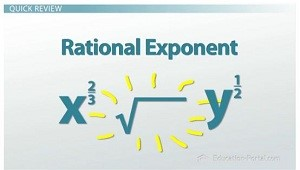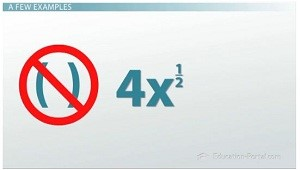# Rational Exponents

#### Video Lesson on Rational Exponents

This lesson video Not available at this time available video coming soon

### Rational Exponents

In this video, learn how to go from a rational exponent to a radical expression and back. No tricks or magic, just good math! We'll review the basics and look at a few examples.

### Quick Review

Now, remember, a rational number is any number that can be written as a fraction. If that's true, what is a rational exponent? A rational exponent is an exponent that is written as a fraction. Here are a couple examples: x^(2/3), y^(1/2).A rational exponent is an exponent that is written as a fraction

You'll notice that the exponent is a fraction. So what is the fraction telling us? The fraction refers to a radical. Let's look at a radical expression.

### Rational to Radical

First, we have the radical symbol. The b is called the index or root number. Inside the radical is the radicand. For our example, x^a is the radicand.

How does that convert to a fractional exponent? Here's what the radical and rational exponent look like together. As you can, see the b in the denominator is the same as the index for the radical. The a in the numerator is the same as the exponent in the radicand.

There are two ways to rewrite a rational exponent: x^(a/b) = bth root of (x^a) = (bth root of x)^a. These all mean the same!

### A Few Examples

Let me show you how this works. So, we have y^2/3 is the same thing as saying the cube root (it's the cube root because we have a 3 here) of y^2. Well, that's the same thing as saying (cube root of y)^2.

Let's look at another one. Here we have the fifth root of x^3. The fifth root is rewritten as the denominator in the rational exponent fraction. The exponent 3 is written as the numerator in the rational exponent fraction. So, the fifth root of x^3 is rewritten as x^(3/5).

Here's the next example: the seventh root of the quantity (2x)^5. How would that look as a rational exponent? The quantity (2x)^(5/7). Remember, the denominator of the rational fraction is the radicand exponent and the numerator is the index number.Without parentheses around the 4x, only the x is raised to the 1/2 power

Mathematicians love to throw a wrench into the system. Let's say I had 4x^(1/2). Do you see the 4x is not in parenthesis like the last one we looked at? Since the 4 and the x are not in parenthesis, only the xis raised to the 1/2 power. The 4 is all by its self. So how would that look as a radical? Since 4 is not raised to a power, it will sit in front of the radical. As far as the radical is concerned, the numerator is 1, so the exponent inside the radicand is 1. The denominator is 2, so this would be our index. When we see a radical symbol without a number in the index, we always assume it's 2.

### Lesson Summary

As you've seen, the fraction tells us we will be rewriting the exponential as a radical expression. The numerator of the fraction is the exponent inside the radicand and the denominator is simply the index.

### About us

EntryTest.com is a free service for students seeking successful career.CAT - College of Admission Tests. All rights reserved. College of Admission Tests Online Test Preparation The CAT Online